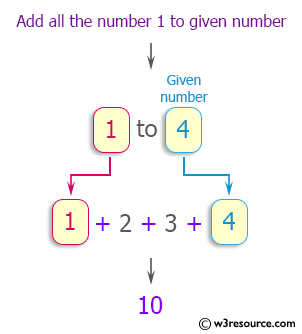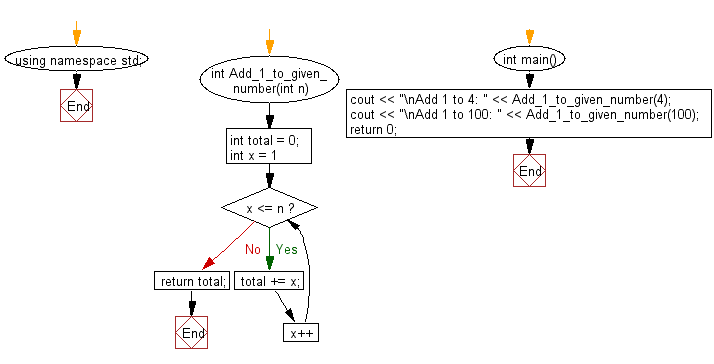﻿ C++ Exercises: Add all the numbers from 1 to a given number - w3resource

# C++ Exercises: Add all the numbers from 1 to a given number

## C++ Basic: Exercise-66 with Solution

Pictorial Presentation:Write a C++ program to add all the numbers from 1 to a given number.

Sample Solution:

C++ Code :

``````#include <iostream>
using namespace std;

int total = 0;

for (int x = 1; x <= n; x++)
{
total += x;
}
}

int main() {

return 0;
}

``````

Sample Output:

```Add 1 to 4: 10
```

Flowchart:C++ Code Editor:

What is the difficulty level of this exercise?

﻿

## C++ Programming: Tips of the Day

Why is there no std::stou?

The most pat answer would be that the C library has no corresponding "strtou", and the C++11 string functions are all just thinly veiled wrappers around the C library functions: The std::sto* functions mirror strto*, and the std::to_string functions use sprintf.

Ref: https://bit.ly/3wtz2qA

We are closing our Disqus commenting system for some maintenanace issues. You may write to us at reach[at]yahoo[dot]com or visit us at Facebook